Conditional Expectations and VariancesHere we look at an important concept that is an extension from Bayes Theorem, which we discussed briefly.

The condition expectation identity says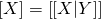The condition variance identity saysHere both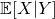andare both functions of Y and are therefore random variables themselves.

With this, we start by considering a random sum of random variables. Let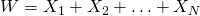where‘s are IID with mean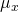and variance, whereis also a random variable, independent of‘s.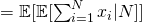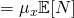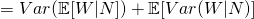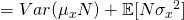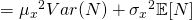Not readable? Change text.